MATH 1
MATH 2
MATH 3
MATH 4
MATH 5
100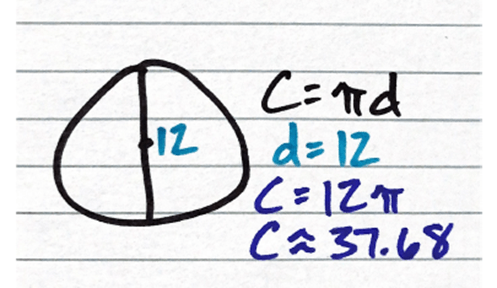What is Circumference?

100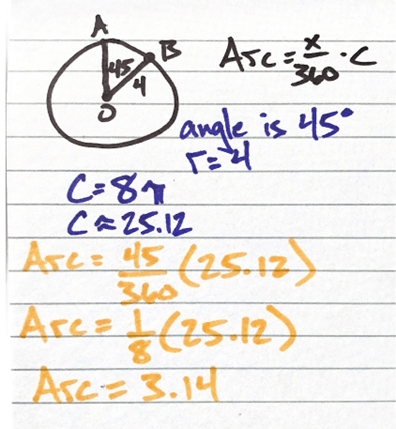What is ARC?

100

The amount of space inside a 2D shape:

rectangle: A=lw
circle: A=πr2

triangle: A=1/2 bh (1/2 base x height)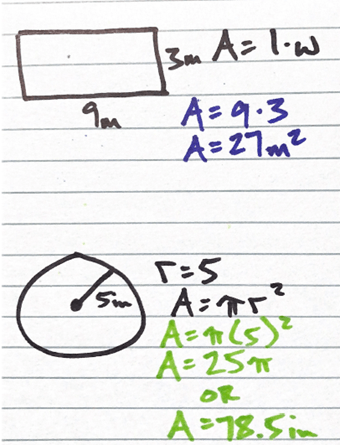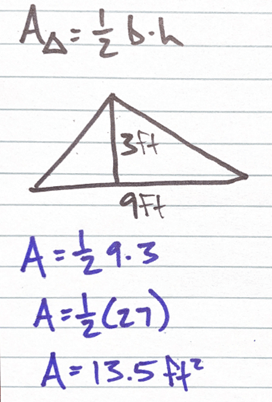What is Area?

100

The top number in a fraction; 1/2

What is the numberator?

100

The bottom number in a fraction; 1/2

What is the denominator?

200

What does PEMDAS stand for?

What is Parentheses, Exponents, Multiplication, Division, Addition, Subtraction.

This is the acronym to help remember the order of operation.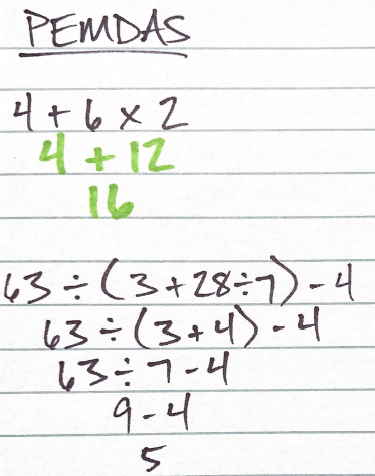200

A “normal” number: any number that can be written as a fraction or terminating or repeating decimals.

Examples:  3/5, 5, etc.

What is a rational number?

200

The relation of two numbers often represented by a fraction.

Examples:  3/5; 3:5

What is a Ratio?

A ratio with units is a rate: 120miles/2hours

200

A ratio in fraction form set equal to another ratio in fraction form.

Example:   2/3 =  10/15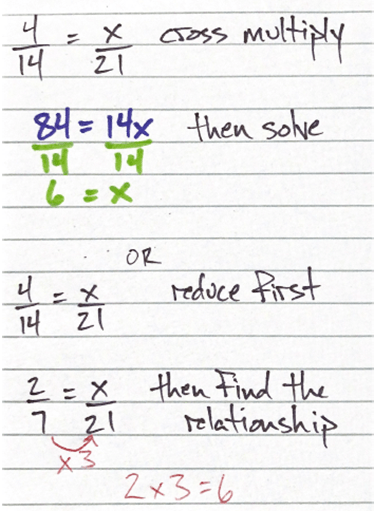What is a proportion?

200

Mean – the average of a group of numbers (add all the numbers and divide that sum by the number of things)

Median – the middle value when numbers are arranged least to greatest

Mode – the number that occurs the most in a group

What are the measures of central tendency?

300

2, 4, 5, 5, 7, 8, 10, 11, 11, 11, 13

Given the set of numbers above: Find the Mean.

What is mean? 7.91

300

Find the mode:

2+4+5+5+7+8+10+11+11+11+13=87
87÷11=7.91

What is mode? 11

The mode is 11 because it occurs the most.
Note: There does not have to be a mode.

300

Find the median:

2+4+5+5+7+8+10+11+11+11+13=87

Note: if there are an even number of values, add the middle two and divide by 2 to get the median.

What is median? 8

300

A letter used to represent an unknown value or quantity. Usually x, y, or z, but can be any letter.

What is a variable?

300

In math, this is one divided by the original number.

Example: the ___ of 4 is  1/4

OR

for fractions, the _____ is the fraction “flipped”

Example: the _____ of 4/5  is 5/4

*Any number multiplied by its _____ equals 1

What is reciprocal?

400

A rate that describes how one quantity changes in relation to another.

This can also be expressed as the slope of a line.

Remember y=mx+b where m is the slope or ___ __ _____.

What is rate of change?

400

A data point that is well outside or separate from other data; an unexpected value.

What is an outlier?

400

The total area of a 3D object’s surface.

Find the area of each surface or face and add them together.

What is surface area?

400

An _____ variable stands on its own; it determines the value of another variable.

What is independent?
400

A _____ variable changes in response to at least one other variable.

What is dependent?
500

A whole number, positive or negative, including zero.

What is an integer?

500

What do these symbols represent?

<, >, ≤, ≥

What are inequality signs?

< Less than
> Greater than
≤ Less than or equal to
≥ Greater than or equal to

500

Arrange the following values from least to greatest:

-3, √9,    7/4,  -2.2,  2

Answer:

-3, -2.2, 7/4, 2, √9

500

To _____ a fraction to a decimal, divide the numerator by the denominator.

2/5 is 2÷5 which gives you 0.4

To _____ a decimal to a percent, multiply the decimal by 100.

Example 0.4 represents 40% or 0.4x100

To _____ a percentage to a decimal, place the percent over 100 and then convert the fraction to a decimal using the steps above.

Example: 25% can be written as 25/100 and then 0.25.
OR
Divide the % by 100 (move the decimal two places to the left): 8.9% = .089

What are conversions?

500

What are inverse operations?

Why/when are they used in math?

Inverse operations are the opposite (adding is the inverse of subtraction; division is the inverse of multiplication) operations.

We use inverse operations most commonly to solve for unknown variables.

Example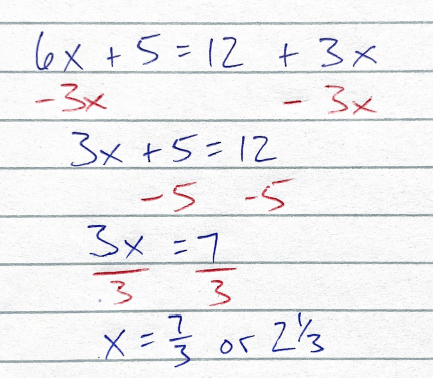First, combine your like variables on one side of the equation. Then perform inverse operations to solve for x (the opposite of what has been done to x).

Click to zoom